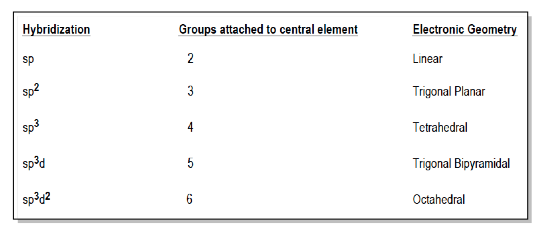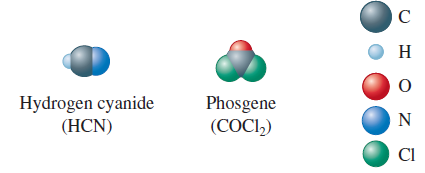# Problem: The space-filling models of hydrogen cyanide and phosgene are shown below.Use the localized electron model to describe the bonding in hydrogen cyanide and phosgene.

###### FREE Expert Solution

Recall: The Localized Electron Model or Valence Bond Theory assumes that all bonds are pair electrons localized between the two atoms. To described the bonding in a molecule, we have to:

1. Draw the Lewis structure of the molecule.
2. Determine the arrangement of electron pairs using the VSEPR model.
3. Determine the hybrid orbitals needed to form the bonds in the molecule.###### Problem Details

The space-filling models of hydrogen cyanide and phosgene are shown below.Use the localized electron model to describe the bonding in hydrogen cyanide and phosgene.